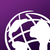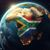# Appending raster properties to table format (csv or text)

1336
4
09-06-2016 02:01 AMOccasional Contributor III

I having trouble to save raster statistics value into csv or text format. Below is my working code. Below code only print the raster statistics value, but i am interested to save the output value to text or csv format.

I appreciate any help you can provide on the crucial bottom code.

Thank you

``````import arcpy
arcpy.env.workspace = r"D:\2008A"
rasterList = arcpy.ListRasters()
for raster in rasterList:
rasterObj = arcpy.Raster(raster)
print raster
bands = arcpy.GetRasterProperties_management(raster, "MEAN")
print "MEAN VALUE: %s" %bands
bands = arcpy.GetRasterProperties_management(raster, "STD")
print "STANDARD DEV: %s" %bands
bands = arcpy.GetRasterProperties_management(raster, "MINIMUM")
print "MIN VALUE: %s" %bands
bands = arcpy.GetRasterProperties_management(raster, "MAXIMUM")
print "Max VALUE: %s" %bands‍‍‍‍‍‍‍‍‍‍‍‍‍‍‍‍‍‍‍‍‍‍‍‍‍‍‍‍``````
Tags (2)
1 Solution

Accepted SolutionsbyMVP Regular Contributor
``````import arcpy
arcpy.env.workspace = r"D:\2008A"
rasterList = arcpy.ListRasters()
csvfile = open(r'D:\2008A\rasters.csv','w')
for raster in rasterList:
rasterObj = arcpy.Raster(raster)
csvfile.write(str(raster) + "\n")
bands = arcpy.GetRasterProperties_management(raster, "MEAN")
csvfile.write("MEAN VALUE: %s \n" %bands)
bands = arcpy.GetRasterProperties_management(raster, "STD")
csvfile.write("STANDARD DEV: %s \n" %bands)
bands = arcpy.GetRasterProperties_management(raster, "MINIMUM")
csvfile.write("MIN VALUE: %s \n" %bands)
bands = arcpy.GetRasterProperties_management(raster, "MAXIMUM")
csvfile.write("Max VALUE: %s \n" %bands)
csvfile.close()‍‍‍‍‍‍‍‍‍‍‍‍‍‍‍‍``````
4 RepliesbyMVP Regular Contributor
``````import arcpy
arcpy.env.workspace = r"D:\2008A"
rasterList = arcpy.ListRasters()
csvfile = open(r'D:\2008A\rasters.csv','w')
for raster in rasterList:
rasterObj = arcpy.Raster(raster)
csvfile.write(str(raster) + "\n")
bands = arcpy.GetRasterProperties_management(raster, "MEAN")
csvfile.write("MEAN VALUE: %s \n" %bands)
bands = arcpy.GetRasterProperties_management(raster, "STD")
csvfile.write("STANDARD DEV: %s \n" %bands)
bands = arcpy.GetRasterProperties_management(raster, "MINIMUM")
csvfile.write("MIN VALUE: %s \n" %bands)
bands = arcpy.GetRasterProperties_management(raster, "MAXIMUM")
csvfile.write("Max VALUE: %s \n" %bands)
csvfile.close()‍‍‍‍‍‍‍‍‍‍‍‍‍‍‍‍``````Occasional Contributor III

FC Basson‌. Thank you very much. Code perfectly workingbyMVP Regular Contributor

You can also add another line break after each raster statistics loop to make it more readable i.e. csvfile.write("\n")Occasional Contributor III

FC Basson‌, yes exactly ,Good suggestion , i have added the line